# 3 digit by 2 Digit Multiplication

### 3 digit by 2 Digit Multiplication Examples

3 digit by 2 Digit Multiplication – Example 1

Find the product.

473 x 32

Explanation

Whenever we multiply a three digit Multiplicand with two digit Multiplier, we first multiply the Multiplicand with ones place of Multiplier, and then multiply Multiplicand with Tens place of the Multiplier.

Step I : Multiply Multiplicand with ones place of Multiplier.

i.e, 473 x 2 = 946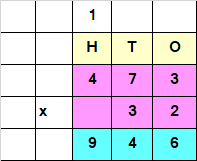Step II : Multiply Multiplicand with tens place of Multiplier.

i.e, 473 x 3 = 1419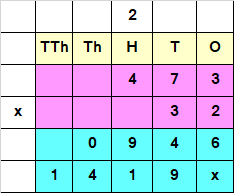Since the Number obtained at Step II, has a Tens value, we add a “0” or “x” at the end of the answer obtained by multiplying Multiplicand with Tens place of the Multiplier.

Step III : Add both the numbers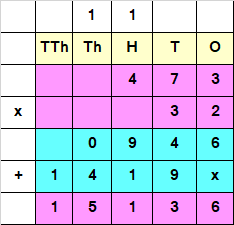Hence, 473 x 32 = 15136

3 digit by 2 Digit Multiplication – Example 2

Find the product.

741 x 45

Explanation

Whenever we multiply a three digit Multiplicand with two digit Multiplier, we first multiply the Multiplicand with ones place of Multiplier, and then multiply Multiplicand with Tens place of the Multiplier.

Step I : Multiply Multiplicand with ones place of Multiplier.

i.e, 741 x 5 = 3705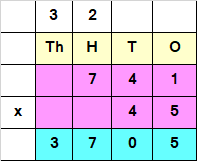Step II : Multiply Multiplicand with tens place of Multiplier.

i.e, 741 x 4 = 2964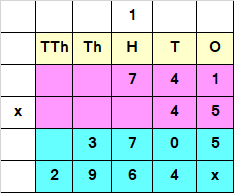Since the Number obtained at Step II, has a Tens value, we add a “0” or “x” at the end of the answer obtained by multiplying Multiplicand with Tens place of the Multiplier.

Step III : Add both the numbers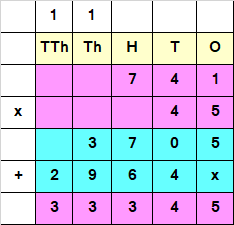Hence, 741 x 45 = 33345